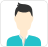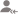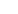true

Find the best tutors and institutes for Engineering Entrance Coaching

Find Best Engineering Entrance Coaching classes

No matching category found.

No matching Locality found.

Outside India?

Search for topicsPost a Lesson

All

All

Lessons

Discussion

Lesson Posted on 04 Mar CBSE/Class 11/Science/Mathematics/Unit-II: Algebra/Complex Numbers and Quadratic Equations CBSE/Class 11/Science/Mathematics/Unit-II: Algebra/Linear Inequalities Exam Coaching/Engineering Entrance Coaching/IIT JEE Coaching/IIT JEE Foundation Course +2 Exam Coaching/Engineering Entrance Coaching/IIT JEE Coaching/IIT JEE Mains Coaching Exam Coaching/Engineering Entrance Coaching/IIT JEE Coaching/IIT JEE Integrated Coaching lessRajan Mittal

I am an Engineer by qualification and a teacher by profession. I have been teaching since 1995. I teach...

Dislike Bookmark

Lesson Posted on 03 Mar CBSE/Class 11/Science/Mathematics/Unit-II: Algebra/Linear Inequalities Exam Coaching/Engineering Entrance Coaching/IIT JEE Coaching/IIT JEE Foundation Course Exam Coaching/Engineering Entrance Coaching/IIT JEE Coaching/IIT JEE Mains CoachingRajan Mittal

I am an Engineer by qualification and a teacher by profession. I have been teaching since 1995. I teach...

Dislike Bookmark

Lesson Posted on 03 Mar CBSE/Class 11 CBSE/Class 11/Science/Mathematics/Unit-II: Algebra/Linear Inequalities Exam Coaching/Engineering Entrance Coaching/IIT JEE Coaching/IIT JEE Foundation CourseRajan Mittal

I am an Engineer by qualification and a teacher by profession. I have been teaching since 1995. I teach...

Dislike Bookmark

Looking for Engineering Entrance Coaching classes

Find best Engineering Entrance Coaching classes in your locality on UrbanPro.

FIND NOW

Lesson Posted on 03 Mar CBSE/Class 11 CBSE/Class 11/Science/Physics/Unit 2-Kinematics/Chapter 4-Motion in a Plane Exam Coaching/NEET-UG Coaching +1 Exam Coaching/Engineering Entrance Coaching/IIT JEE Coaching/IIT JEE Foundation Course lessRajan Mittal

I am an Engineer by qualification and a teacher by profession. I have been teaching since 1995. I teach...

Dislike BookmarkRajan Mittal

I am an Engineer by qualification and a teacher by profession. I have been teaching since 1995. I teach...

Dislike BookmarkRajan Mittal

I am an Engineer by qualification and a teacher by profession. I have been teaching since 1995. I teach...

Dislike BookmarkRajan Mittal

I am an Engineer by qualification and a teacher by profession. I have been teaching since 1995. I teach...

This video gives explanatory solution to NEET 2018 Q.1 For physics section
Dislike Bookmark

Answered on 19 Apr Exam Coaching/Engineering Entrance CoachingRahul P Sah

4 years of teaching experience in math physics and computer application

You can enter into the field of data science or data analyst, AI and ML, and earn a handsome package. Also, python is a trending language, so that you can get community support for at least the upcoming two decades.
Dislike BookmarkPriyanka Rana

IITian Mathematics Teacher with 4 years of experience

There is absolutely no problem if you join Coaching for the IIT JEE exam, but you should try a few sample question papers of FIITJEE and Akash entrance exams before that. That will give you a hint of what is the difficulty level and will increase your interest in the subjects as well.
Dislike Bookmark

Looking for Engineering Entrance Coaching classes

Find best Engineering Entrance Coaching classes in your locality on UrbanPro.

FIND NOWRavi kota

I am a Pro-active, responsible and result-oriented Math trainer with a strong passion for student improvement...

How to prove if points are Collinear in coordinate geometry? Collinear points definition: Three or more points that lie on a same straight line are called collinear points. Consider a straight line L in the above Cartesian coordinate plane formed by x axis and y axis. This straight line L is... read more

How to prove if points are Collinear in coordinate geometry?

Collinear points definition:

• Three or more points that lie on a same straight line are called collinear points.
• Consider a straight line L in the above Cartesian coordinate plane formed by x axis and y axis.
• This straight line L is passing through three points A, B and C whose coordinates are (2, 4), (4, 6) and (6, 8) respectively.
• {We may also say, alternatively, that the three points A (2, 4), B (4, 6) and C (6, 8)are lying on a same straight line L}
• Three or more points which lie on a same straight line are called collinear points.

How to find if three points are collinear?:

• There are two methods to find if three points are collinear.
• One is slope formula method and the other is area of triangle method.
• Slope formula method to find that points are collinear.
• Three or more points are collinear, if slope of any two pairs of points is same.
• With three points A, B and C, three pairs of points can be formed, they are: AB, BC and AC.
• If Slope of AB = slope of BC = slope of AC, then A, B and C are collinear points.

Example

Show that the three points A (2, 4), B (4, 6) and C (6, 8) are collinear.

Solution:

• If the three points A (2, 4), B (4, 6) and C (6, 8) are collinear, then
• slopes of any two pairs of points will be equal.
• Now, apply slope formula to find the slopes of the respective pairs of points:
• Slope of AB = (6 – 4)/ (4 – 2) = 1,
• Slope of BC = (8 – 6)/ (6 – 4) = 1, and
• Slope of AC = (8 – 4) /(6 – 2) = 1
• Since slopes of any two pairs out of three pairs of points are same, this proves that A, B and C are collinear points.
• Area of triangle to find if three points are collinear.
• Three points are collinear if the value of area of triangle formed by the three points is zero.
• Apply the coordinates of the given three points in the area of triangle formula. If the result for area is zero, then the given points are said to be collinear.
• First of all, recall the formula for area of a triangle formed by three points.

It isIn the formula above, the two vertical bars enclosing the variables represent a determinant.

Let us apply the coordinates of the above three points A, B and C in the determinant formula above for area of a triangle to check if the answer is zero.Since the result for area of triangle is zero, therefore A (2, 4), B (4, 6) and C (6, 8) are collinear points.

Dislike Bookmark

UrbanPro.com helps you to connect with the best Engineering Entrance Coaching classes in India. Post Your Requirement today and get connected.

Overview

Questions 1.6 k

Lessons 159

Total Shares101,435 Followers

## Engineering Entrance Coaching in:

x

X

### Looking for Engineering Entrance Coaching Classes?

Find best tutors for Engineering Entrance Coaching Classes by posting a requirement.

• Post a learning requirement
• Get customized responses
• Compare and select the best### Looking for Engineering Entrance Coaching Classes?

Find best Engineering Entrance Coaching Classes in your locality on UrbanProUrbanPro.com is India's largest network of most trusted tutors and institutes. Over 55 lakh students rely on UrbanPro.com, to fulfill their learning requirements across 1,000+ categories. Using UrbanPro.com, parents, and students can compare multiple Tutors and Institutes and choose the one that best suits their requirements. More than 7.5 lakh verified Tutors and Institutes are helping millions of students every day and growing their tutoring business on UrbanPro.com. Whether you are looking for a tutor to learn mathematics, a German language trainer to brush up your German language skills or an institute to upgrade your IT skills, we have got the best selection of Tutors and Training Institutes for you. Read more